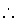Next: Bibliography Up: Small Projects Previous: Ordinary Differential Equations

# A Scheduling Program

We want to develop a program to schedule a project, decomposed in a set of tasks. Each task has successor tasks and a length. A high level view of the whole program has this layout:

• Construct the data structure defining the project; this could be made reading from a external file, but for the sake of simplicity, we will store it as a fact in the program. The rest of the program works exactly in the same way if this structure is built from a external source.
• Some checks are made regarding the integrity of the data--i.e., we want the data describing the project to make sense.
• After that, the project data is used to generate the constraints which model the relationships among the tasks.
• Finally, the total time in the project is minimized, and the results are written to standard output.

sched(P):-
project(P, Td),
check_data(Td),
close_structure(Dict),
write_results(Dict).


The definition of the project is (for this example) just a fact with relates a project name (the non-imaginative a) with a list of tasks, which define the initial and final tasks, and for each task, its name (an atom), its length, and the tasks which depend on it (atoms, again). This list will be used to built the constraint network and a dictionary where information about the tasks will be stored. The final task has, associated to it, an absolute limit for the project span.

project(a, [initial(a), task(a,0,[b,c,d]), task(b,1,[e]),


Checking the correctness of the data is one of the less elegant parts of the program. Each element in the list is checked to make sure that it defines the initial task, the final task, or an intermediate task. For each of them, we will also check that atoms appear where are expected, and that numbers appear where task lengths are expected.

check_data([]).
check_data([T|Ts]):-
check_datum(T),
check_data(Ts).

check_datum(Initial):-
Initial = initial(Name),
check_atoms([Name], Initial), !.
check_datum(Final):-
Final = final(Name, Limit),
check_atoms([Name], Final),
check_number(Limit, Final), !.
check_datum(What):-
write_atoms(['Found ' ,What, ' (unknown).']).

check_atoms([], _Where).
check_atoms([A|As], Where):-
check_atom(A, Where),
check_atoms(As, Where).

check_atom(A, _Where):- atom(A), !.
check_atom(A, Where):-
write_atoms(['Found ', A, ' in ', Where, ', expecting atom.']).

check_number(N, _Where):- number(N), !.
check_number(N, Where):-
write_atoms(['Found ', N, ' in ', Where, ', expecting number.']).

write_atoms([]):- nl.
write_atoms([A|As]):-
write(A),
write_atoms(As).


The process of building the constraints actually makes two things: it sets up the constraints themselves, but it also constructs a dictionary which relates the task (the Key of each dictionary entry) with the task's< Start and Length (the Value associated to the Key). This is implemented using an open list (a list whose tail ends in a free variable), so that only one argument has to be used for the dictionary. In the case of a larger project, it might be advantageous replacing it by a binary sorted tree. The predicate lookup/4 is the only entry point for the dictionary: it retrieves and, in case of non-existence, adds new items.

lookup(Task, Start, Len, Dict):-

insert(Key, Value, [pair(Key, ThisValue)|_Rest]):- !, Value = ThisValue.
insert(Key, Value, [_OtherPair|Rest]):- insert(Key, Value, Rest).


As a utility predicate, and to make clearer the final printing of the list of tasks, close_structure/1 closes the dictionary, i.e., it will make the final variable of the list a [].

close_structure([]):- !.
close_structure([_|R]):- close_structure(R).


The core of the program is the constraint generation. For each item in the project definition we add the corresponding constraint. Tasks are related one to each other through constraints which are actually put on the variables associated to the tasks names in the dictionary. The name of the final task is returned, so that the minimization predicate can use it to reduce the length of the project as much as possible. The actions taken for creating the constraints are:

• The initial task is searched for in the dictionary, and simultaneously the associated Start time is set to zero.
• The final task is looked up in the dictionary, and its end time is bounded using the limit which was associated to the project.
• For every other task (among which the first and last task can also appear), the successor tasks are scheduled to be started after the current task is finished. Their names are looked up in the dictionary, and their start time are forced to be greater than the current tasks' finish time.

build_constraints([], _FinalTask, Dict).

lookup(Name, Start, Len, Dict),
End = Start .+. Len,
previous(Succ, End, Dict).
lookup(Name, 0, _Len, Dict).
le(End, Limit),
End = Start .+. Len,
lookup(Name, Start, Len, Dict).

previous([], _End, Dict).


Minimizing is made naïvely, which is enough for this application: the start of the last task (which has length zero) is forced to be at its minimum. In other cases special builtin predicates will have to be used.

minimize(FinalTask, Dict):-
glb(Start, Start).


Finally, writing the results takes advantage of the structure of the dictionary, and dumps it in a more readable form:

write_results([]).
write_results(Ps).

' starts at ', L, '.']).
lt(L, U),
' can start from ', L, ' to ', U, '.']).


And a query, with the results, is:

?- sched(a).

Task a with length 0 starts at 0.
Task b with length 1 can start from 0 to 1.
Task c with length 2 starts at 0.
Task d with length 3 can start from 0 to 2.
Task e with length 4 starts at 2.
Task f with length 1 can start from 3 to 5.
Task g with length 0 starts at 6.Next: Bibliography Up: Small Projects Previous: Ordinary Differential Equations
MCL
1998-12-03